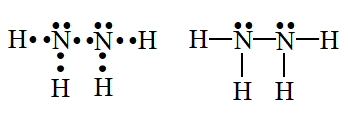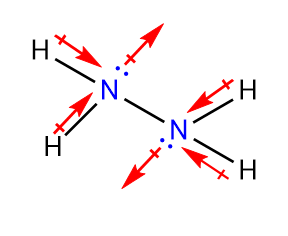# N2H4 Lewis Structure, Geometry, Hybridization, and PolarityN2H4 is the chemical formula for hydrazine which is an inorganic compound and a pnictogen hydride. It is also known as Diazane or Diamine or Nitrogen hydride and is alkaline. It is highly toxic and mostly used as a foaming agent in the preparation of polymer foams.

Also, it is used in pharmaceutical and agrochemical industries.

In this article, we will study the lewis structure of N2H4, geometry, hybridization, and its lewis structure.

Contents

## Lewis Structure of N2H4

Lewis dot diagram or electron dot structure is the pictorial representation of the molecular formula of a compound along with its electrons that are represented as dots.

These structures are named after American chemist Gilbert Newton Lewis who introduced them in 1916.

Lewis structures illustrate the chemical bonding between different atoms of a molecule and also the number of lone pairs of electrons present in that molecule.

These are the representation of the electronic structure of the molecule and its atomic bonding where each dot depicts an electron and two dots between the atoms symbolize a bond.

The Lewis structure of N2H4 is given below.We already know that only the valence electrons of an atom participate in chemical bonding to satisfy the octet for that atom. In the case of N2H4 nitrogen has five electrons while hydrogen has only one valence electron.

Here, this must be noted that the octet rule does not apply to hydrogen which becomes stable with two electrons.

Therefore, each nitrogen atom forms a single bond with two hydrogen atoms and the other nitrogen atom, thus, satisfying the octet rule for all the participating atoms.

### Drawing Lewis Structure of N2H4

The following steps should be followed for drawing the Lewis diagram for hydrazine:

• First of all, we will have to calculate the total number of valence electrons present in the molecule.

Here, Nitrogen is a group 15th element and therefore, has 5 electrons in its outermost shell while hydrogen is the first element of the periodic table with only one valence electron.

Adding the valence electrons of all the atoms to determine the total number of valence electrons present in one molecule N2H4.

Nitrogen = 5 Valence electrons; for 2 Nitrogen atoms, 2 * 5 = 10

Hydrogen = 1 valence electron; for 4 Hydrogen atoms, 4 * 1 = 4

Therefore, the total number of valence electrons in N2H4 = 14• Post this we will try to draw the rough sketch of the Lewis diagram by placing the atoms in a definite pattern connected with a single bond.

This is meant to give us the estimate about the number of electrons that remain unbounded and also the number of electrons further required by any atom to complete their octet.

• Here, you may ask the reason for this particular sequence for nitrogen and hydrogen molecules in N2H4 molecule i.e. why are nitrogen atoms placed at the center even when nitrogen is more electronegative than hydrogen.

Actually, the Nitrogen atom requires three electrons for completing its octet while the hydrogen atom only requires placing nitrogen atoms at the center brings symmetry to the molecule and also makes sharing of electrons amongst different atoms easier.

• It is clear from the above structure that after sharing one electron each with two hydrogen atoms and the other nitrogen atom the octet of both the nitrogen atoms is satisfied as they also have a lone pair of electrons each.

• Therefore, the final structure for the N2H4 molecule looks like this:• The accuracy of the Lewis structure of any molecule can be determined by calculating the formal charge on that molecule.

The formal charge is a hypothetical concept that is calculated to evaluate the stability of the derived lewis structure.

• For maximum stability, the formal charge for any given molecule should be close to zero. It is calculated individually for all the atoms of a molecule.

The formula for calculation of formal charge is given below:

Formal Charge (FC) = [Total no. of valence e– in Free State] – [Total no. of non-bonding e– – 1/2 (Total no. of bonding e–)]• Now, calculating the formal charge for the N2H4 molecule:

For the Nitrogen atom, the Total number of valence electrons in free state = 5

Total number of nonbonding electrons = 2

Total number of bonding electrons = 6

Therefore, Formal charge on nitrogen atom = 5 – 2 – ½(6)

= 0

For Hydrogen atom, Total number of valence electrons in free state = 1

Total number of non-bonding electrons = 0

Total number of bonding electrons = 2

Therefore, Formal charge on nitrogen atom = 1 – 0 – ½(2)

= 0

• Hence, the total formal charge on the N2H4 molecule becomes zero indicating that the derived structure is stable and accurate.

## Geometry of N2H4The postulates described in the Valence Shell Electron Pair Repulsion (VSEPR) Theory are used to derive the molecular geometry for any molecule.

As per this theory, the electrons of different atoms inside a molecule tend to arrange themselves as far apart as possible so that they face the least inter-electronic repulsion.

All the electrons inside a molecule including the lone pairs exert inter-electronic repulsion. However, the maximum repulsion force exists between lone pair-lone pair as they are free in space.

Therefore, the geometry of a molecule is determined by the number of lone pairs and bonding pairs of electrons as well as the distance and bond angle between these electrons.

Also, the inter-electronic repulsion determines the distortion of bond angle in a molecule.

Now, to understand the molecular geometry for N2H4 we will first choose a central atom.

The VSEPR theory assumes that all the other atoms of a molecule are bonded with the central atom. As both the Nitrogen atoms are placed at the center of the Lewis structure any one of them can be considered the central atom.

Hence, in the case of N2H4, one Nitrogen atom is bonded with two Hydrogen atoms and one nitrogen atom.

Taking into account the VSEPR theory if the three bonded electrons and one lone pair of electrons present on the Nitrogen atom are placed as far apart as possible then it must acquire trigonal pyramidal shape.

Looking at the molecular geometry of N2H4 through AXN notation in which A is the central atom, X denotes the number of atoms attached to the central atom and N is the number of lone pairs.

Hence, for the N2H4 molecule, this notation can be written as AX3N indicating that it has trigonal pyramidal geometry. The electron geometry for the N2H4 molecule is tetrahedral.

The following table represents the geometry, bond angle, and hybridization for different molecules as per AXN notation:The bond angle here is 109.5° as stated in the table given above. The molecular geometry for the N2H4 molecule is drawn as follows:

## Hybridization of N2H4

Hybridization is the process of mixing one or more atomic orbitals of similar energy for the formation of an entirely new orbital with energy and shape different from its constituent atomic orbitals.

This concept was first introduced by Linus Pauling in 1931.

The hybrid orbitals so formed due to intermixing of atomic orbitals are named after their basic orbitals i.e. their names indicate the orbitals involved in their formation.

For example, the sp3 hybrid orbital indicates that one s and 3 p-orbitals were involved in its formation.

The hybridization of any molecule can be determined by a simple formula that is given below:

Hybridization = Number of sigma (σ) bond on central atom + lone pair on the central atom

Now, calculating the hybridization for N2H4 molecule using this formula:

Here, No. of sigma bonds = 3

And, No. of lone pairs = 1

Hence, Hybridization = 3 + 1

= 4

Therefore, the hybridization for the N2H4 molecule is sp3.

Also, as mentioned in the table given above a molecule that has trigonal pyramidal shape always has sp3 hybridization where the one s and three p-orbitals are placed at an angle of 109.5°.## Polarity of N2H4

The existence of two opposite charges or poles in a molecule is known as its polarity.

The reason for the development of these charges in a molecule is the electronegativity difference that exists between its constituent atoms.

In the case of the N2H4 molecule we know that the two nitrogen atoms are in the same plane and also there is no electronegativity difference between these two atoms, hence, the bond between them is non-polar.

The polarity of the N2H4 molecule arises due to the electronegativity difference between the Nitrogen and hydrogen atoms.

Comparing the two Nitrogen atoms in the N2H4 molecule it can be noted that they have the same number of hydrogen atoms as well as lone pairs of electrons.

However, the hydrogen atoms attached to one Nitrogen atom are placed in the vertical plane while the hydrogen atoms attached to the other Nitrogen atom are located in the horizontal plane.

This results in developing net dipole moment in the N2H4 molecule.

Also, the shape of the N2H4 molecule is distorted due to which the dipole moment of different atoms would not cancel amongst themselves.

The net dipole moment for the N2H4 molecule is 1.85 D indicating that it is a polar molecule.## Properties of N2H4

The important properties for N2H4 molecule are given in the table below:

 Name Hydrazine Chemical Formula N2H4 Color Colorless Appearance Fuming, oily liquid Molecular weight 32.0452 g/mol Density 1.021 g/cm3 Boiling Point 114 °C Melting Point 2 °C Dipole Moment 1.85 D Hybridization sp3 Bond Angle 109.5° Solubility Miscible in water Refractive Index 1.46044

## Uses of N2H4

A few of the important uses of hydrazine are given below:

• It is used in the preparation of polymer foams

• It is used as the storable propellant for space vehicles as it can be stored for a long duration.

• It is used as a precursor for many pesticides.

• It is used in pharmaceutical and agrochemical industries.

• It is used for electrolytic plating of metals on glass and plastic materials.

• In cooling water reactors it is used as a corrosion inhibitor.

• It is used as an inorganic solvent.

## Conclusion

The Lewis structure for the N2H4 molecule is:The formal charge on this Lewis structure is zero indicating that this is the authentic structure.

The molecular geometry for the N2H4 molecule is trigonal pyramidal and the electron geometry is tetrahedral.

The hybridization of N2H4 is sp3.

The dipole moment for the N2H4 molecule is 1.85 D.

Hope you understand the lewis structure, geometry, hybridization, and polarity of N2H4. In case, you still have any doubt, please ask me in the comments.

Happy Learning! and check out my more interesting posts.